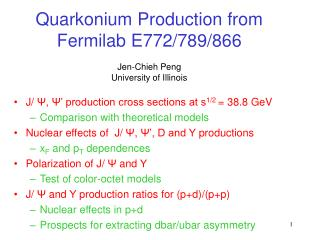DownloadDownload PresentationQuarkonium Production from Fermilab E772/789/866 Jen-Chieh Peng University of Illinois

# Quarkonium Production from Fermilab E772/789/866 Jen-Chieh Peng University of Illinois

Télécharger la présentation## Quarkonium Production from Fermilab E772/789/866 Jen-Chieh Peng University of Illinois

- - - - - - - - - - - - - - - - - - - - - - - - - - - E N D - - - - - - - - - - - - - - - - - - - - - - - - - - -
##### Presentation Transcript

1. Quarkonium Production from Fermilab E772/789/866Jen-Chieh PengUniversity of Illinois • J/ Ψ, Ψ’ production cross sections at s1/2 = 38.8 GeV • Comparison with theoretical models • Nuclear effects of J/ Ψ, Ψ’, D and Υ productions • xF and pT dependences • Polarization of J/ Ψ and Υ • Test of color-octet models • J/ Ψ and Υ production ratios for (p+d)/(p+p) • Nuclear effects in p+d • Prospects for extracting dbar/ubar asymmetry

2. (E605/772/789/866) Meson East Spectrometer Open-aperture Closed-aperture Beam-dump (Cu) J/Ψ J/Ψ Ψ’ σ(J/ψ) ~ 15 MeV σ(J/ψ) ~ 150 MeV σ(J/ψ) ~ 300 MeV

3. J/Ψ production cross sections E789 data p + Cu (beam dump) → J/Ψ + x S1/2 = 38.8 GeV Calculations using Color Evaporation Model and CTEQ5L parton distributions g-g total q-qbar No nuclear effects included Nuclear dependence taken into account

4. J/Ψ and Ψ’ cross sections E789 data p + Au → J/Ψ (Ψ’) + x (open aperture) S1/2 = 38.8 GeV Large discrepancy between the observed J/Ψ (Ψ’) cross sections and the calculations of color-singlet model

5. Nuclear effects of Quarkonium productions p + A at s1/2 = 38.8 GeV E772 data σ(p+A) = Aασ(p+N) Strong xF - dependence Nuclear effects scale with xF, not x2 (should be checked at RHIC/LHC energies)

6. Nuclear effects of open-charm production p + A → D + x at s1/2 = 38.8 GeV E789 open-aperture, silicon vertex + dihadron detection h+h- mass spectrum (after vertex cut) No nuclear effect for D production (at xF ~ 0) D0→ K-π+ Need to extend the measurements to large xF region

7. Comparison between the J/Ψ and Ψ’ nuclear effects p + A → J/Ψ or Ψ’ at s1/2 = 38.8 GeV α(xF) is largely the same for J/Ψ and Ψ’ (except at xF ~ 0 region) ‘Universal’ behavior for α(pT) (similar for J/Ψ, Ψ’; weak s1/2 dependence)

8. PT - broadening for D-Y, J/Ψ and Υ Extract <PT2> from fits to data Δ<PT2> for J/Ψ is larger than for D-Y Similar behavior for J/Ψ and Υ

9. Preliminary A = 1.2 A = 1.35 A = 2 A = 2 Preliminary E866 - J/Y Nuclear dependence even for Deuterium/Hydrogen Nuclear dependence in deuterium seems to follow the systematics of larger nuclei, but with an effective A smaller than two. From fits to E866/NuSea p + Be, Fe, W data:

10. Polarization of J/Ψ and Υ E866 p + Cu → J/Ψ + x (beam dump) s1/2 = 38.8 GeV (hep-ex/030801) Typical dimuon mass spectrum for various xF, pT, cosθ bins dσ/dΩ ~ 1 + λcos2θ (extraction of λ for various xF, pT bins)

11. Polarization of J/Ψ in p + Cu Collision dσ/dΩ ~ 1 + λ cos2θ (λ=1: transversely polarized, λ = -1: longitudinally polarized λ = 0, unpolarized) E866 data λ is small, but nonzero λ becomes negative at large xF No strong pT dependence for λ hep-ex/030801

12. Polarization of Υ(1S),Υ(2S+3S) p + Cu →Υ + x (E866 beam-dump data) Dimuon mass spectrum λ for D-Y, Υ(1S), Υ(2S+3S) Decay angular distributions Υ(1S) D-Y is transversely polarized Υ(1S) is slightly polarized (like J/Ψ) Υ(2S+3S) is transversely polarized! Need to measure Ψ’ polarization Υ(2S+3S)

13. đ/ū flavor asymmetry and quarkonium production σDY(p+d) / 2σDY(p+p) ~ (1 + đ/ū) / 2 đ / ū đ - ū đ / ū at x > 0.15 need to be better determined (Future Fermilab E906 experiment with 120 GeV proton)

14. Is quarkonium production also sensitive to đ/ū asymmetry? Both gluon-gluon fusion and q-qbar annihilation contribute Color-evaporation model calculation for p+p at 800, 120 and 50 GeV (CTEQ5L PDF) total g-g q-qbar 800 GeV 120 GeV 50 GeV CEM predicts dominance of q-qbar annihilation at lower beam energies

15. σJ/Ψ(p+d) / 2σJ/Ψ(p+p) at lower beam energies is sensitive to đ/ū asymmetry CEM calculations for σJ/Ψ(p+d)/2σJ/Ψ(p+p) at 800, 120 and 50 GeV 800 GeV 120 GeV 50 GeV E866 preliminary ū ≠ đ ū = đ Future J/Ψ measurements at E906 (and at JHF) could provide interesting information on đ/ū asymmetry

16. đ/ū asymmetry from Υ production? σΥ(p+d)/2σΥ(p+p) CEM calculation (CTEQ5L) total q-qbar gg E866 preliminary Future J/Ψ data could provide much improved statistics

17. Future prospects • J/Ψ A-dependence at negative xF will be available from RHIC and LHC • Open-charm A-dependence data at large xF is crucial for understanding medium effects • Polarization of quarkonium remains a mystery. Ψ’ polarization data from E866 are being analyzed • J/Ψ production at lower beam energies (120 GeV Main-Injector and 50 GeV JHF) could provide interesting new information on their production mechanisms as well as đ/ū flavor asymmetry